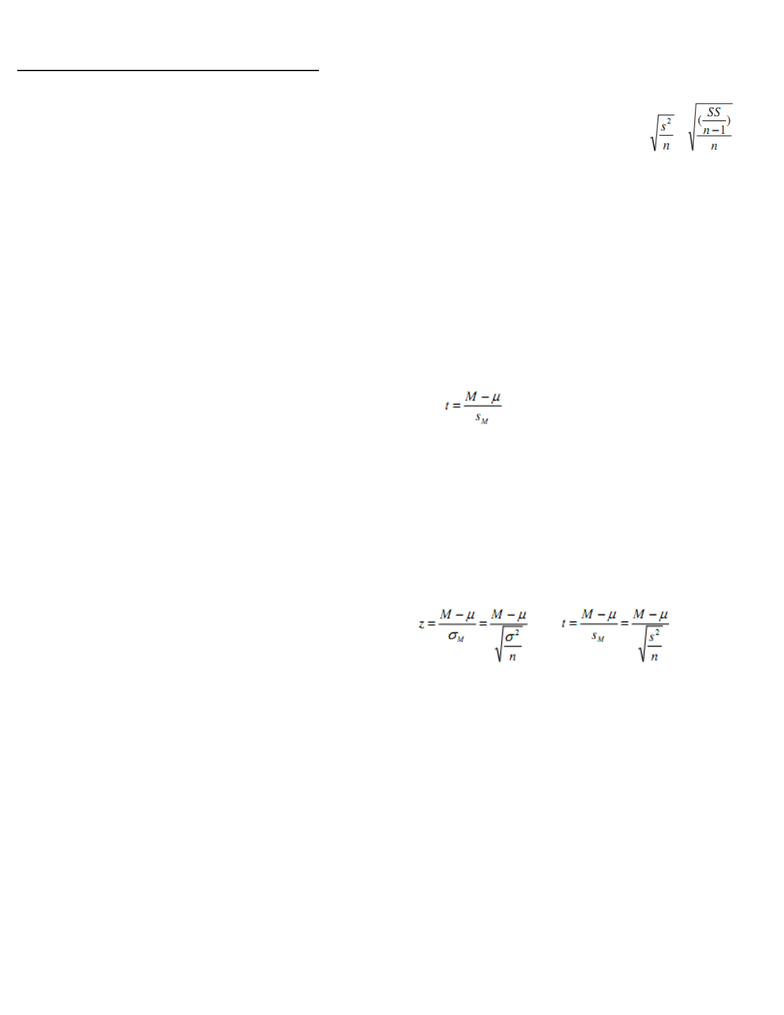# PSY201H1 Lecture Notes - Lecture 9: Variance, Standard Error, Test Statistic

42 views4 pagesPSY201 Lecture 9; Nov. 17, 2011
Introduction to the t Statistic
The t Statistic: An Alternative to z
When hypothesis testing:
Use a sample mean M to approximate the
pop mean µ.
Standard error, σM, measures how well M
approximates µ
σM = σ/√n
Then compare M to µ by computing the
relevant z-score test statistic
z = (M - µ)/ σM
Use to determine whether the obtained
dif is greater than expected by chance
by looking at unit normal table (for a
normal distribution)
Like z-score, t statistic allows researchers to use
sample data to test hypotheses about an
unknown µ
Doesn’t require any knowledge of the pop
σwhich we typically don’t know
So can be used to test hypotheses about a
completely unknown pop, ie:
Both µ & σ unknown
Only available info about pop comes
from sample
All required for a hypothesis test with t is a
sample & a reasonable hypothesis about µ
When we don’t haveσcan estimate it using the
sample variability
Much like estimating µ using M
Can use t statistic when determining whether
treatment causes a change in µ
Sample is obtained from the pop treatment is
As usual, if resulting sample M is significantly
different from original µ, can conclude that
treatment has a significant effect
Like before, hypothesis test attempts to decide
btwn:
Ho: Is it reasonable that the discrepancy
btwn M & µ is simply due to sampling error
and not result of treatment effect?
H1: Is the discrepancy btwn M & µ more than
expected by sampling error alone?
ie. Is M significantly different from µ
Critical 1st step for t statistic hypothesis test:
Calculate exactly how much dif btwn M & µ is
reasonable to expect
But since σ is unknown, impossible to
compute standard error of M (σM = σ/√n) as
done w z-scores
t statistic requires to use sample
data’s variance, s2 to compute
estimated standard error, sM
sM = s/√n
Rmbr s2 = SS/(n-1)
s = √(SS/df)
sM used more in terms of sample variance, since
provides accurate & unbiased estimate of the
pop variance σ2
Estimated sample error = =
Rmbr must know sample mean before
computing sample variance, so there is a
restriction on sample variability
Only n - 1 scores are independent & free to
vary
n - 1 = degrees of freedom (df) for sample
variance
t statistic (like the z-score) forms a ratio:
Numerator: Obtained dif btwn M
hypothesized µ
Denominator: Estimated standard error
(measures how much dif expected by
chance)
A large value for t (a large ratio) indicates
obtained difference btwn data & hypothesis >
expected if treatment has no effect (ie. is just
error)
W large sample, df large, so estimation is
very good
So t statistic will be very similar to z-
score
W small samples, df small, so t statistic
provides relatively poor estimate of z
Can think of the t statistic as "estimated zscore."
;
Just like we can make a distribution of z-scores,
we can make a t distr
Complete set of t values computed for every
possible random sample for a specific
sample size n, or specific degrees of freedom
df
As df approaches infinity, t distr
approximates normal distr
How well it approximates normal distr
depends on df
ie. There’s a “family” of t distrs so there’s a
dif sampling distr of t for each possible df
Larger n larger n – 1 better the t distr
approximates the normal distr
W small values for df t distr flatter & more
spread out than a normal distr
Unlock document

This preview shows page 1 of the document.
Unlock all 4 pages and 3 million more documents.